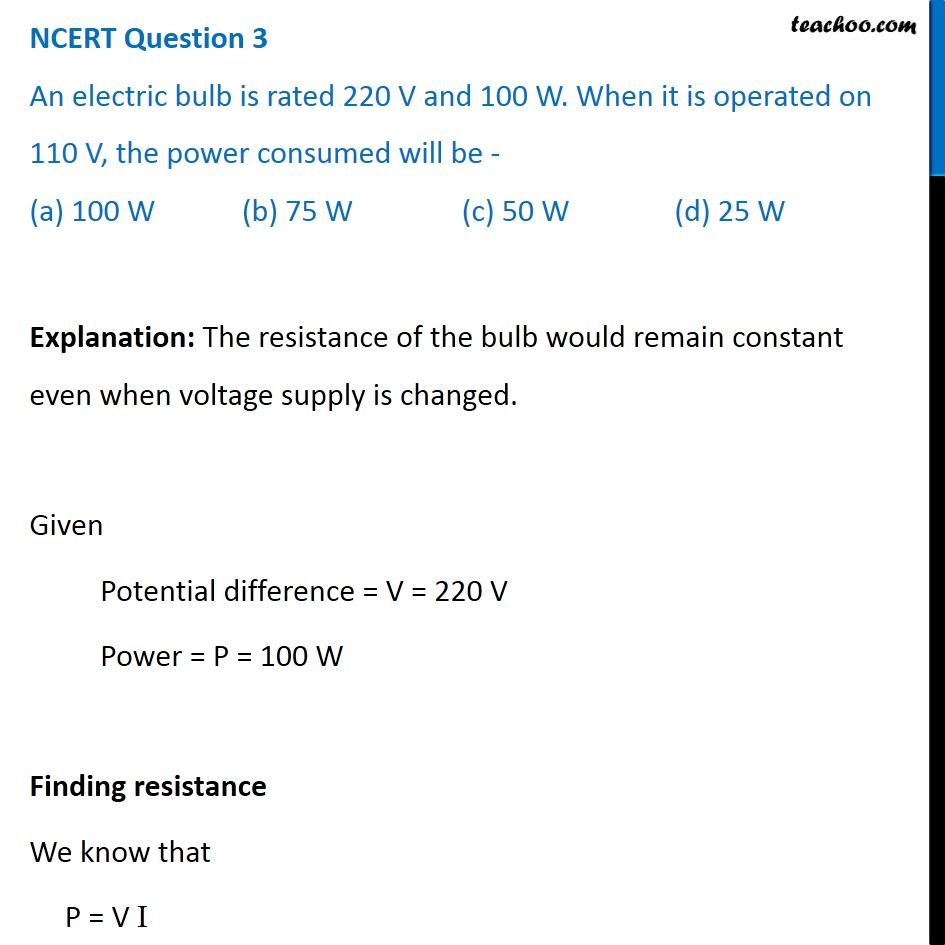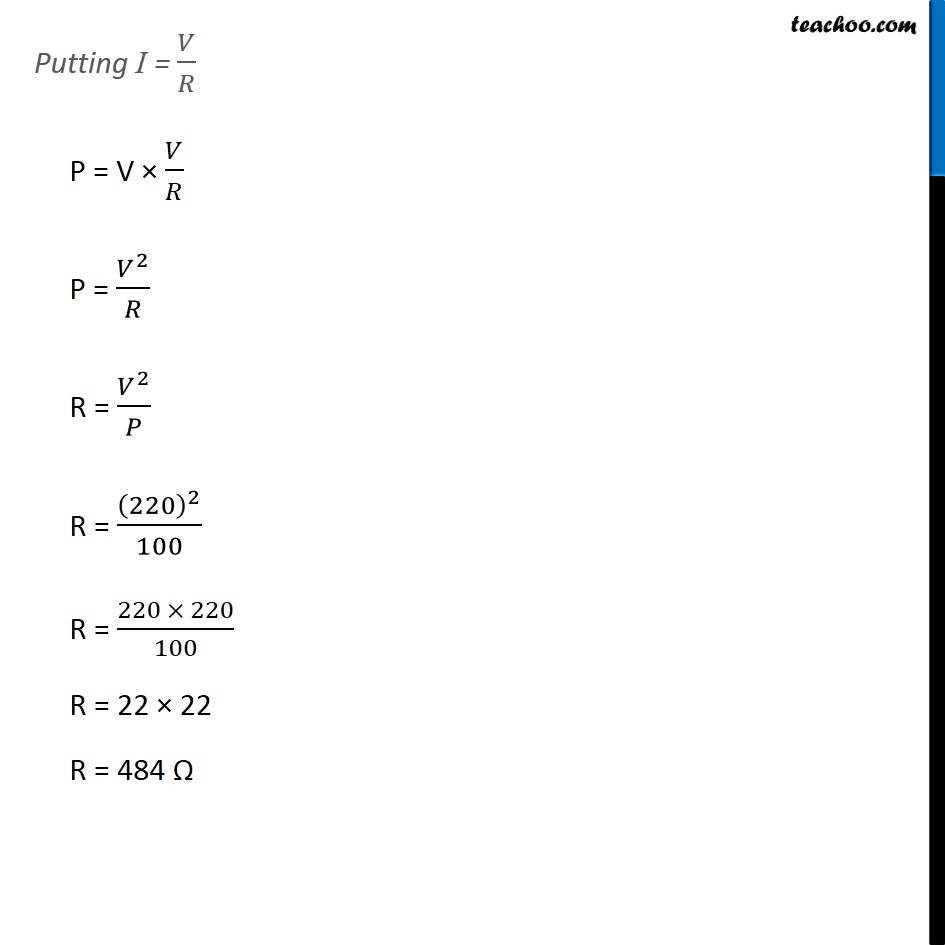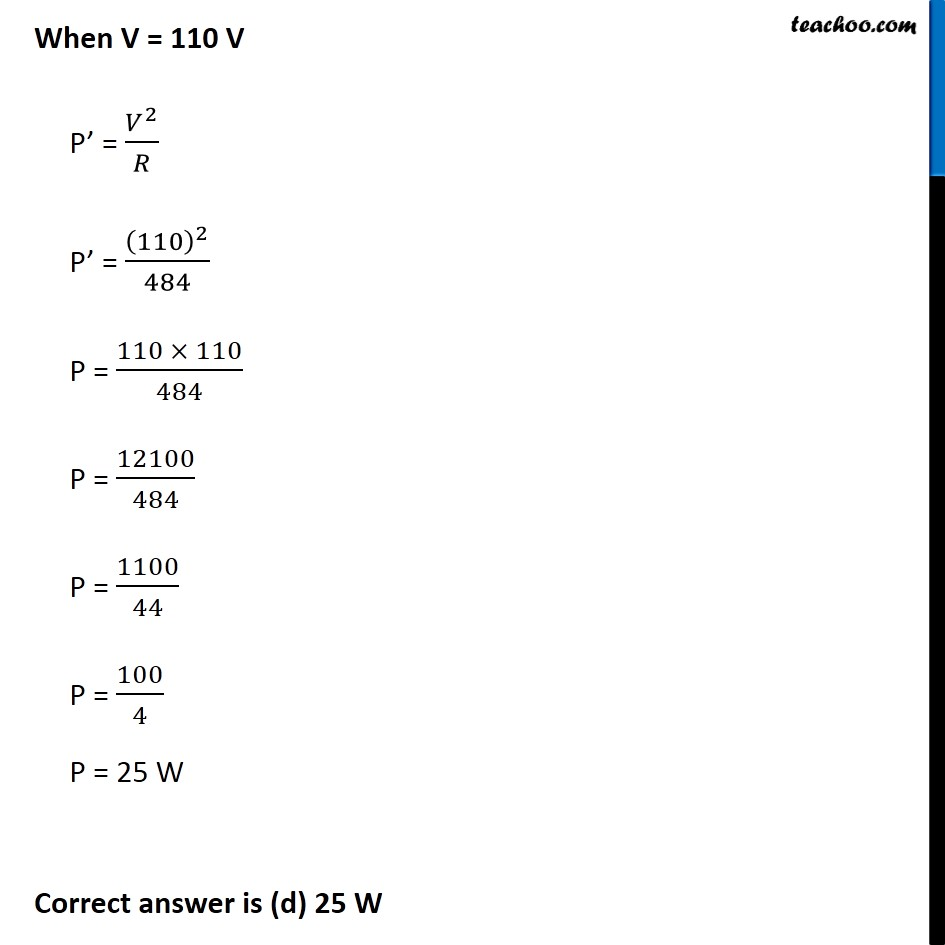NCERT Questions

Class 10
Chapter 12 Class 10 - Electricity

## (a) 100 W (b) 75 W (c) 50 W (d) 25 WGet live Maths 1-on-1 Classs - Class 6 to 12

### Transcript

NCERT Question 3 An electric bulb is rated 220 V and 100 W. When it is operated on 110 V, the power consumed will be - (a) 100 W (b) 75 W (c) 50 W (d) 25 W Explanation: The resistance of the bulb would remain constant even when voltage supply is changed. Given Potential difference = V = 220 V Power = P = 100 W Finding resistance We know that P = V I Putting I = 𝑉/𝑅 P = V × 𝑉/𝑅 P = 𝑉^2/𝑅 R = 𝑉^2/𝑃 R = (220)^2/100 R = (220 × 220)/100 R = 22 × 22 R = 484 Ω When V = 110 V P’ = 𝑉^2/𝑅 P’ = (110)^2/484 P = (110 × 110)/484 P = 12100/484 P = 1100/44 P = 100/4 P = 25 W Correct answer is (d) 25 W